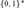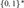Home

# BROUWER’S FAN THEOREM AND CONVEXITY

## Abstract

In the framework of Bishop’s constructive mathematics we introduce co-convexity as a property of subsets B of${\left\{ {0,1} \right\}^{\rm{*}}}$ , the set of finite binary sequences, and prove that co-convex bars are uniform. Moreover, we establish a canonical correspondence between detachable subsets B of${\left\{ {0,1} \right\}^{\rm{*}}}$ and uniformly continuous functions f defined on the unit interval such that B is a bar if and only if the corresponding function f is positive-valued, B is a uniform bar if and only if f has positive infimum, and B is co-convex if and only if f satisfies a weak convexity condition.

Hide All

# BROUWER’S FAN THEOREM AND CONVEXITY

## Metrics

### Full text viewsFull text views reflects the number of PDF downloads, PDFs sent to Google Drive, Dropbox and Kindle and HTML full text views.

Total number of HTML views: 0
Total number of PDF views: 0 *Loading metrics...

### Abstract viewsAbstract views reflect the number of visits to the article landing page.

Total abstract views: 0 *Loading metrics...

* Views captured on Cambridge Core between <date>. This data will be updated every 24 hours.

Usage data cannot currently be displayed